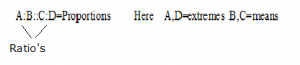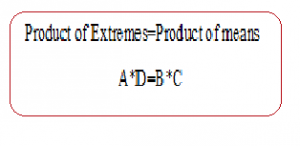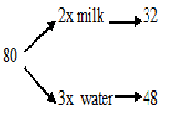# TRICKS TIPS AND SHORTCUTS TO SOLVE PROBLEMS ON PROPORTIONS

Hi Bankersdaily Aspirants,

### “We will receive not what we idly wish for but what we justly earn. Our rewards will always be in exact proportion to our service.”

As you all know we have started a new Learn Series to help with your preparations for various exams like IBPS PO , IBPS RRB Scale I officer , IBPS RRB Office Assistant , IBPS Clerk , IBPS SO , SBI PO , SBI Clerk , SBI SO and many other exams in this type. Nevertheless this series will also contribute you all the tips and tricks to every miscellaneous sections and also all the important topics which are asked in the exams. If you haven’t looked at the Learn series , Please Click the below link to learn the new concepts.

Aspirants,As the Quotes implies there is Proportionality everywhere we go,So today we are going to discuss on Proportions.Before knowing about Proportions have a look on Ratio’s for better understanding.

## PROPORTIONS:

### The equality of two ratios is called proportions.

Proportion has basically 5 types,Questions asked in IBPS Exams are nowadays with high difficulty,So, to solve all the difficult problem we should know how to solve the basic problems.Here we have covered all the basic Problem and its types and Shortcut method for solving all the basic Problems

That is:### Rule:If  X=KY, K=constant then X is directly proportional toY

XY=K, then X is inversely proportional to Y.

### FINDING NUMBER OF PERSON:

These type of question in Proportionality are the basic type and we can solve these type of  problems by two methods

1.Method 1:Solving by equations

2.Method 2:Shortcut Method

#### 1.A sum of Rs.312 is to be distributed among 100 boys and girls such that if each boy gets Rs.3.60 and each girl gets Rs.2.40 then find how many number of boys in the class?

Explanation:

Let there be X boys in the class and Y girls in the girls

X+Y=100

3.6X+2.4Y=312

By solving 1 & 2 we get X=60 and Y=40

The number of boys present in the class=60

Shortcut Method:

 Boys Girls 3.60 2.40 3 2

100*(3/5)=60.The number of Boys=60

### BASED  ON ADDITION/SUBTRACTION OF QUANTITY:

#### 1In a 80 litre of solution containing milk and water in the ratio 2:3.How much milk should be added to the solution so that the new ratio becomes 4:1?

Explanation:The new ratio is 4:1 that means only water is added to the solution which means that the water in the solution remains constant in the new ratio also

Therefore  1x=48litre

4x=192 litre where as the solution already contain 32 litre therefore we should add 160litres of milk

#### 2.In a 60 litres mixture of milk and water the ratio of milk and water is 7:5.How much water should be added in the mixture so that the ratio of milk to water becomes 5:7?

Explanation:
 Mixture 60 Milk Water 7x=35 5x=25

Milk is not added and kept as it is so 5x=35        x=7 there by 7x=7*7=49

Already there is 25litres of water in the mixture so additionally  we need to add 24 litres of water

## BASED ON EQUALITY:

If the original ratio a:b

Duplicate Ratio:a²:b²

Sub Duplicate Ratio:  √a:√b

Triplicate Ratio:  a³:b³

Sub Triplicate Ratio:a^(1/3):b^(1/3)

#### 1.If twice a is equal to 3b and that is equal to 5 times of c.Then find a:b:c?

Explanation:

LCM of 2,3,5=30

a:b:c=30/2:30/3:30/5

=15:10:6

Explanation:

√a:√b=2/3

a:b=4/9

a³:b³=64/729

#### 3.A hound pursues a hare and takes 6 leaps foe every 9 leaps of the hare,but 3 leaps of the hound are equal to 5leaps of the hare.Compare the rates of the hound and the hare?

Explanation:

Given:

 Hound Hare 6L 9L

3Hound=5Hare

Hound=(5/3)Hare

Rates of Hound:Rates of Hare

6*(5/3)L   :  9L

10:9

### BASED  ON PROPORTION:

#### 1.If the height of the person is directly proportional to the square root of the age,if the height of the person is 5ft and his age be 36,then find what is the height of the person  whose age is 64?

Explanation:

Here,the height is directly Proportional to age then

 Height Age 5 6 X 8

Height=(5*8)/6=20/3

## #.5  TYPE 5:

### BASED ON TWO RATIO’S:

1.If a mixture containing milk and water in the ratio 9:5 and another mixture containing milk  and water in the ratio 4:3 the mixture in the 2 container are equal and the third quantity a new mixture is formed.Find the ratio of milk and water in the new mixture?

Explanation:
 1st container(14x) 2nd Container(7x) Milk Water Milk Water 9x 5x 4x 3x 1st container(14x) 2nd Container is equal to 1st container as per question)2*7x=(14x) 9x 5x 8x 6x

9x+8x  5x + 6x

17 : 11

### You may also like to read the following articles:

#### TRICKS, TIPS & SHORTCUTS IN NUMBER RANKING – REASONING SECTION

TIPS TRICKS AND SHORTCUT METHOD TO SOLVE BOATS AND STREAMS